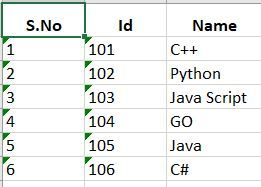Related Articles
Writing to Excel Sheet Using EPPlus in C#
• Last Updated : 14 Feb, 2020

EPPlus is a very helpful open-source 3rd party DLL for writing data to excel. EPPlus supports multiple properties of spreadsheets like cell ranges, cell styling, charts, pictures, shapes, comments, tables, protection, encryption, pivot tables, data validation, conditional formatting, formula calculation, etc.

First of all install EPPlus using Packet Manager console by writing the following command:

```Install-Package EPPlus
```

Let’s see how to create and write to an excel-sheet using C#.

 `using` `System;``using` `System.IO;``// The following to two namespace contains``// the functions for manipulating the``// Excel file ``using` `OfficeOpenXml;``using` `OfficeOpenXml.Style;`` ` `class` `Program``{``    ``static` `void` `Main(``string``[] args)``    ``{``        ``var` `Articles = ``new``[]``        ``{``                ``new` `{``                    ``Id = ``"101"``, Name = ``"C++"``                ``},``                ``new` `{``                    ``Id = ``"102"``, Name = ``"Python"``                ``},``                ``new` `{``                    ``Id = ``"103"``, Name = ``"Java Script"``                ``},``                ``new` `{``                    ``Id = ``"104"``, Name = ``"GO"``                ``},``                ``new` `{``                    ``Id = ``"105"``, Name = ``"Java"``                ``},``                ``new` `{``                    ``Id = ``"106"``, Name = ``"C#"``                ``}``            ``};`` ` `        ``// Creating an instance``        ``// of ExcelPackage``        ``ExcelPackage excel = ``new` `ExcelPackage();`` ` `        ``// name of the sheet``        ``var` `workSheet = excel.Workbook.Worksheets.Add(``"Sheet1"``);``         ` `        ``// setting the properties``        ``// of the work sheet ``        ``workSheet.TabColor = System.Drawing.Color.Black;``        ``workSheet.DefaultRowHeight = 12;`` ` `        ``// Setting the properties``        ``// of the first row``        ``workSheet.Row(1).Height = 20;``        ``workSheet.Row(1).Style.HorizontalAlignment = ExcelHorizontalAlignment.Center;``        ``workSheet.Row(1).Style.Font.Bold = ``true``;``     ` `        ``// Header of the Excel sheet``        ``workSheet.Cells[1, 1].Value = ``"S.No"``;``        ``workSheet.Cells[1, 2].Value = ``"Id"``;``        ``workSheet.Cells[1, 3].Value = ``"Name"``;`` ` `        ``// Inserting the article data into excel``        ``// sheet by using the for each loop``        ``// As we have values to the first row ``        ``// we will start with second row``        ``int` `recordIndex = 2;``         ` `        ``foreach` `(``var` `article ``in` `Articles)``        ``{``            ``workSheet.Cells[recordIndex, 1].Value = (recordIndex - 1).ToString();``            ``workSheet.Cells[recordIndex, 2].Value = article.Id;``            ``workSheet.Cells[recordIndex, 3].Value = article.Name;``            ``recordIndex++;``        ``}``         ` `        ``// By default, the column width is not ``        ``// set to auto fit for the content``        ``// of the range, so we are using``        ``// AutoFit() method here. ``        ``workSheet.Column(1).AutoFit();``        ``workSheet.Column(2).AutoFit();``        ``workSheet.Column(3).AutoFit();`` ` `        ``// file name with .xlsx extension ``        ``string` `p_strPath = ``"H:\\geeksforgeeks.xlsx"``;``         ` `        ``if` `(File.Exists(p_strPath))``            ``File.Delete(p_strPath);`` ` `        ``// Create excel file on physical disk ``        ``FileStream objFileStrm = File.Create(p_strPath);``        ``objFileStrm.Close();`` ` `        ``// Write content to excel file ``        ``File.WriteAllBytes(p_strPath, excel.GetAsByteArray());``        ``//Close Excel package``        ``excel.Dispose();``        ``Console.ReadKey();`` ` `    ``}``}`

Output:In this program, we are taking static values for the Articles data but in real-time, we can use database call and foreach loop for iteration of each record.

My Personal Notes arrow_drop_up